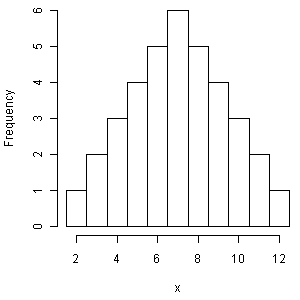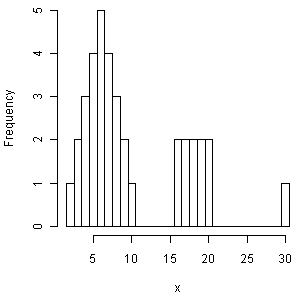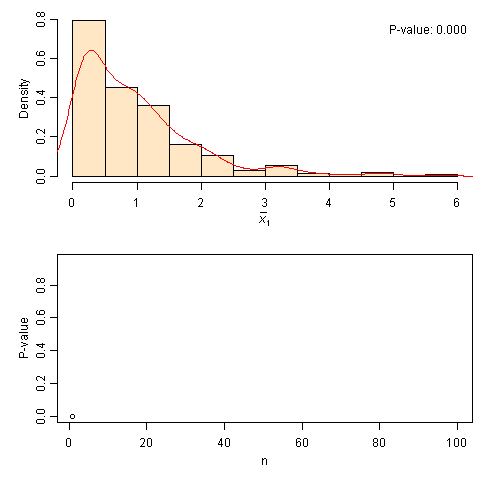# 二、中心极限定理的模拟

## 1、对称的钟形曲线不代表正态分布

data a;
do x1=1 to 6;
do x2=1 to 6;
output;
end;
end;  /*模拟掷骰子两次，生成36行数据*/
data a;
set a;
x=sum(x1,x2);
proc univariate data=a noprint;
var x;
histogram/normal (mu=est sigma=est);
run;


x = rowSums(expand.grid(1:6, 1:6))
hist(x, breaks = seq(min(x) - 0.5, max(x) + 0.5, 1), main = "")两次掷骰子得到的结果之和的分布

d = c(1, 2, 3, 4, 5, 15)
x = rowSums(expand.grid(d, d))
hist(x, breaks = seq(min(x) - 0.5, max(x) + 0.5, 1), main = "")6个数字中2个样本之和的分布

## 3、给出拟合好坏的指标

if (!require(animation)) install.packages("animation")
library(animation)
ani.options(interval = 0.1, nmax = 100)
par(mar = c(4, 4, 1, 0.5))
clt.ani()中心极限定理模拟：从指数分布到正态分布

# 脚注

symbol;
goptions ftext= ctext= htext=;

%macro a(n);
data rv;
retain _seed_ 0;
do _i_ = 1 to &n;
binom1 = ranbin(_seed_,&n,0.8); /*ranbin函数返回以seed、n、0.8为参数的二项分布随机变量*/
output;
end;
drop _seed_ _i_;
run;
%mend;

%a(100);

proc univariate data=rv noprint;
var binom1;
histogram/normal( mu=est sigma=est);
inset normal ;
run;


R代码：

a = function(n) hist(rbinom(n, n, 0.8))